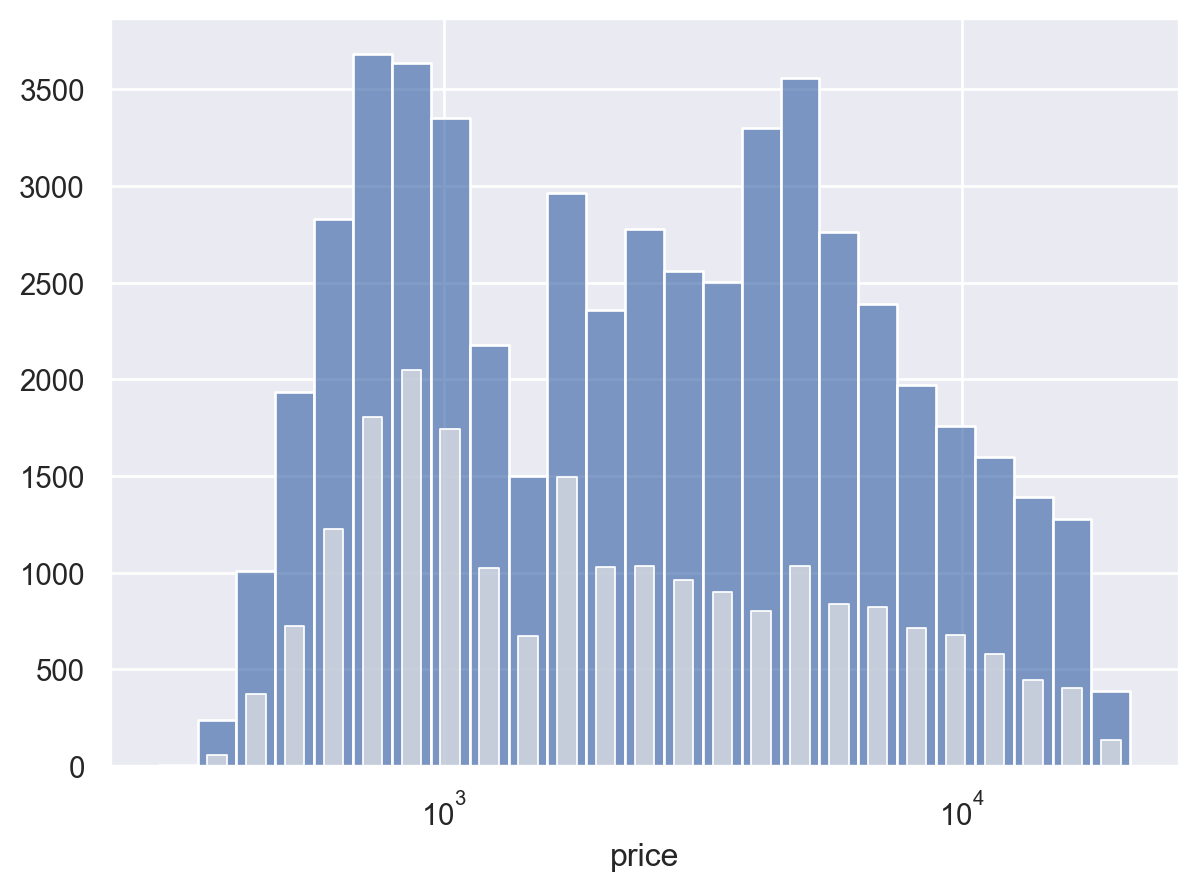# seaborn.objects.Bars#

class seaborn.objects.Bars(artist_kws=<factory>, color=<'C0'>, alpha=<0.7>, fill=<True>, edgecolor=<rc:patch.edgecolor>, edgealpha=<1>, edgewidth=<auto>, edgestyle=<'-'>, width=<1>, baseline=<0>)#

A faster bar mark with defaults more suitable histograms.

This mark defines the following properties:

`Bar`

A bar mark drawn between baseline and data values.

Examples

This mark draws bars between a baseline and a value. In contrast to `Bar`, the bars have a full width and thin edges by default; this makes this mark a better choice for a continuous histogram:

```p = so.Plot(diamonds, "price").scale(x="log")
```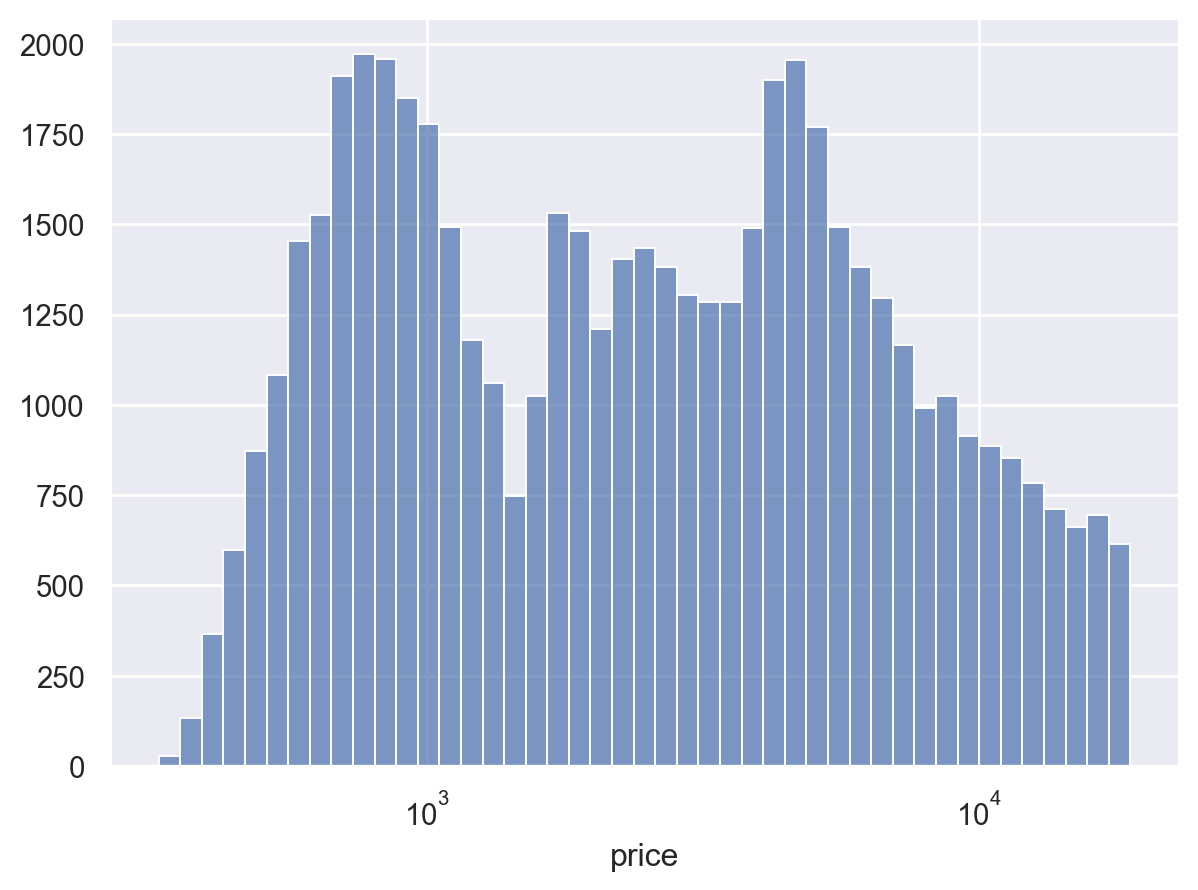When mapping the color or other properties, bars will overlap by default; this is usually confusing:

```p.add(so.Bars(), so.Hist(), color="cut")
```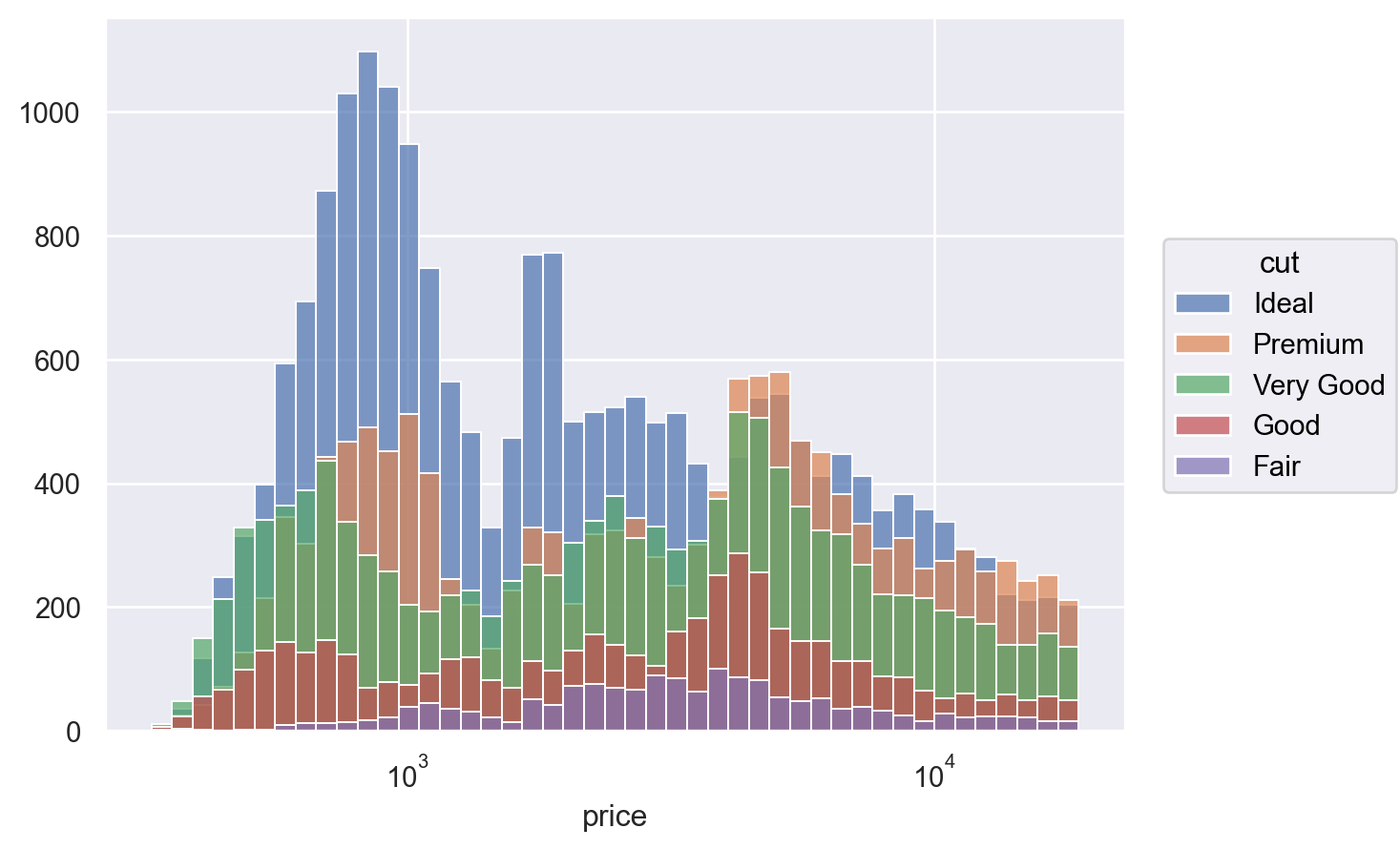Using a move transform, such as `Stack` or `Dodge`, will resolve the overlap (although faceting might often be a better approach):

```p.add(so.Bars(), so.Hist(), so.Stack(), color="cut")
```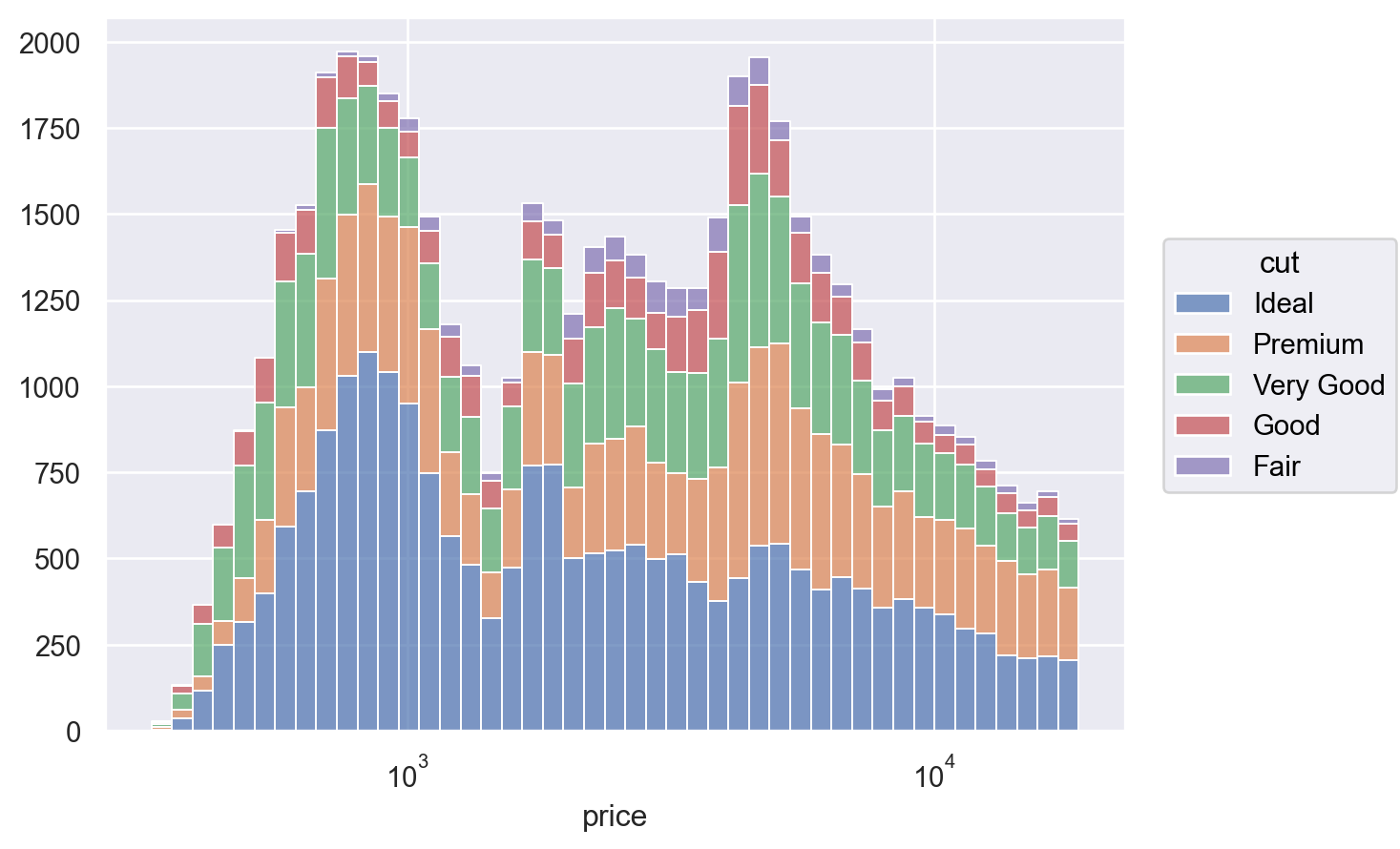A number of different properties can be set or mapped:

```p.add(so.Bars(edgewidth=0), so.Hist(), so.Stack(), alpha="clarity")
```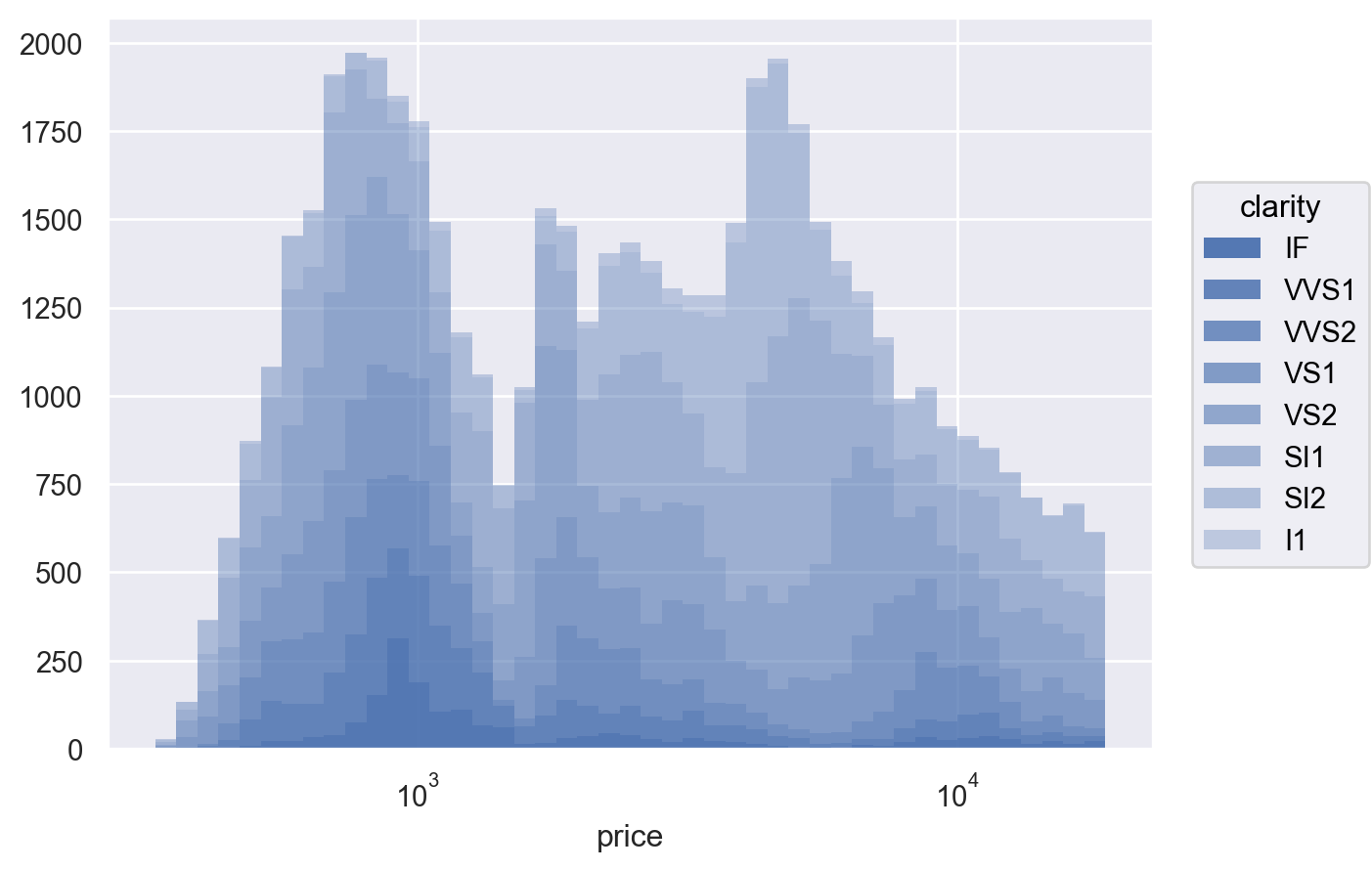It is possible to draw unfilled bars, but you must override the default edge color:

```p.add(so.Bars(fill=False, edgecolor="C0", edgewidth=1.5), so.Hist())
```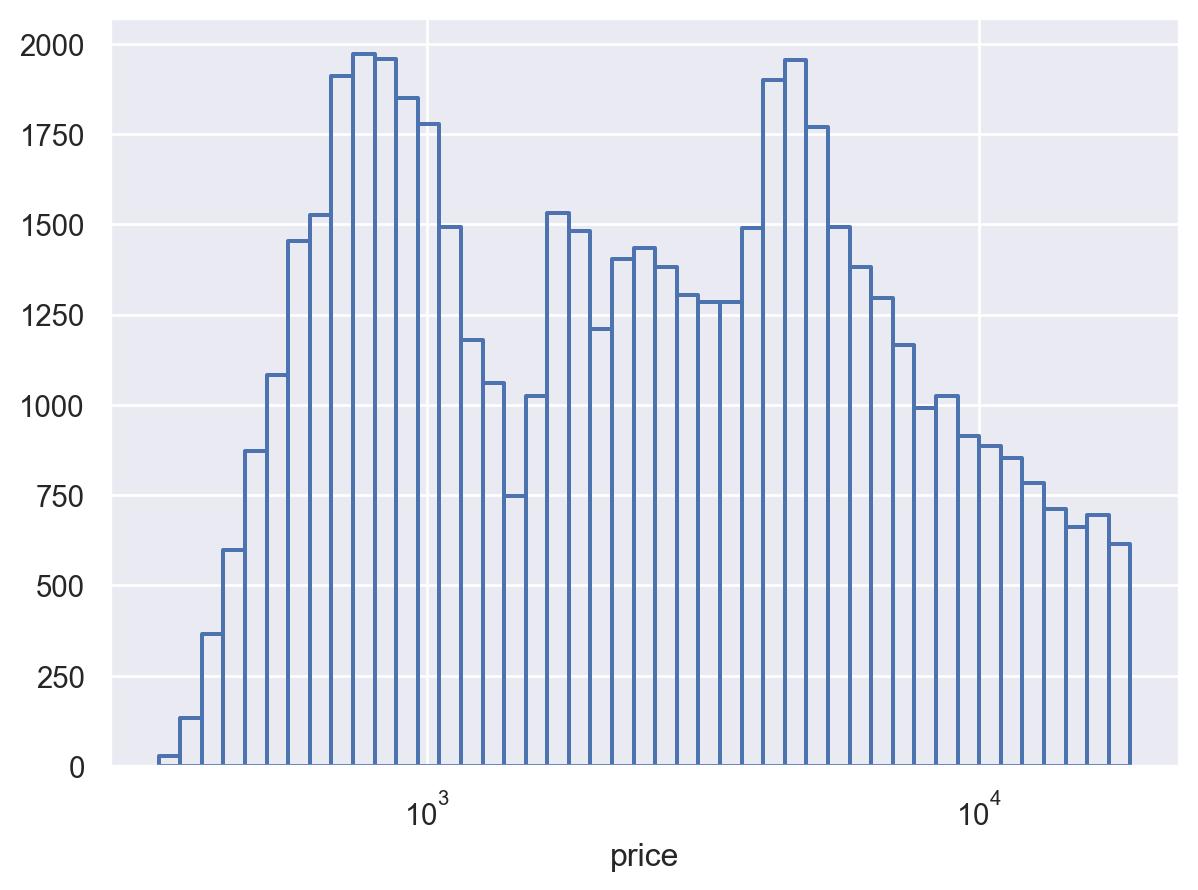It is also possible to narrow the bars, which may be useful for dealing with overlap in some cases:

```hist = so.Hist(binwidth=.075, binrange=(2, 5))
(